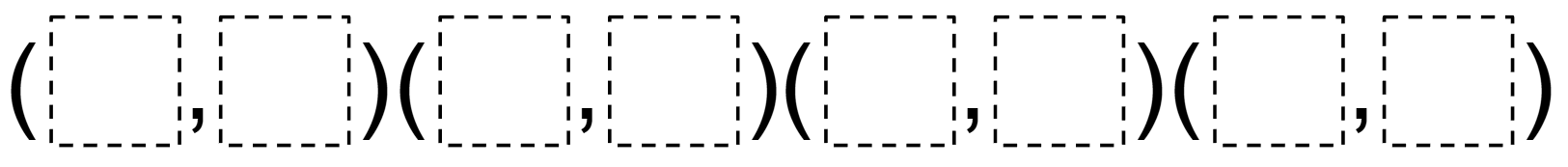Home > Grade 6 > Geometry > Area of a Quadrilateral on a Coordinate Plane

# Area of a Quadrilateral on a Coordinate Plane

Directions: Using the digits 0 to 9 at most one time each, fill in the blanks to create a quadrilateral with an area of 16 square units.### Hint

What happens if two ordered pairs have the same x-value?
What happens if two ordered pairs have the same y-value?

### Answer

One solution is (1,2), (3,0), (5,6), (7,4). Note: If you change the range of integers to be -9 to 9, then the question is also aligned to standard 6.NS.6b.

Source: Daniel Luevanos

## Subtracting Multi-Decimals

Directions: Use the digits 1 to 9, at most one time each, to fill in …

### 7 comments

1.I am not sure that I agree that this problem is aligned with 6th grade standards. I believe that given the coordinates, 6th graders should be able to compose a larger rectangle by drawing 4 right triangles surrounding the graphed quadrilateral. The resultant rectangle would have coordinates with same first or last coordinates and its area could be found. Students could also find the area of the right triangles and subtract those from the larger rectangle to find the area of the original graphed rectangle. This would correlate with CCSS 6.G.3 I might also change the challenge to allow use of integers between -9 and 9 and allowing them to repeat which would also align with G.1 and G.3 Do you have any other suggestions or is my understanding faulty?
I really like this site and the problems.
Thank you.

•Great suggestion. I can see how this question could be aligned to 6.G.3. If you did change the range of numbers to be -9 to 9, then the question can also be aligned to 6.NS.6b. Glad you like the questions on the website too! This is one of my favorite websites!

•C Farmer, if you are saying it would be very difficult for a 6th grader (pre-Pythagorean Thm) to construct the rectangle to meet the conditions, but that it is reasonable for them to come up with a way to figure out the area for this particular solution, then I agree. Or maybe you’re suggesting giving them the surrounding square and having them carve off right triangles?

2.My sixth grade students successfully reached an answer to this problem today! They are immensely proud of themselves, and I am immensely proud of their perseverance — this problem spanned multiple math periods for us. They propose these coordinates: (3,6), (8,5), (7,2), (1,4). Thank you for challenging my students!

•Daniel Luevanos

This is so awesome, Kimberly! Thank you for sharing! Great work, kids!

3.When we have plotted these points on a Cartesian Plane we have gotten the rectangle, however why does the formula for square units of area not seem to be able to be applied. We have a length of 4 but only a width of 2 units?? Please help!

•I get a 2 unit by 4 unit rectangle, as well, when I plot the ordered pairs for the given answer. This would give an area of 8 square units. Am I missing something?